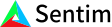1. Bob Anderson
×
IntuitiveML

## Intuition: Artificial Neural Networks

May 30, 2020

Artificial Neural Networks consist of layers of nodes where every node represents a function on its input. Similarly, every connection in this network represents some coefficient of our function. When dealing with neural networks, we call these coefficients weights. Additionally, there is an activation function that takes in the sum of these weighted inputs and modifies it in some way. All together, the output for a single node is just the activation function of the sum of the weighted inputs. Every layer in our network just adds another layer of nesting to the function we are building. So when we create an artificial neural network (ANN), all we are doing is creating a complicated function on the inputs. So how does creating this function help solve our problem?

First off, we assume there is some underlying function that maps the data we have to the solution. This is pretty reasonable. For example, given any picture, there should be some function that says whether or not there is a face in that picture.

So given our neural network, how do we modify it to so that it will learn a function to map the input to the associated output? How do we train the network? To train our neural network, the neural network looks at every example in the data set, and if it produced the incorrect answer, we slightly adjust the weights of the network so that the output of the function is slightly closer to the desired output. Then, by repeating this process over and over for lots of examples, the network will slowly learn a function that produces better and better results over the data set.

One question that remains is that given our initial function, how do we choose the coefficients? Rather, when we create neural networks, how do we choose the starting weights? Well, since we already know that we will change the weights to better represent the data, it doesn’t matter what they are initially, so most of the time we just make them random.